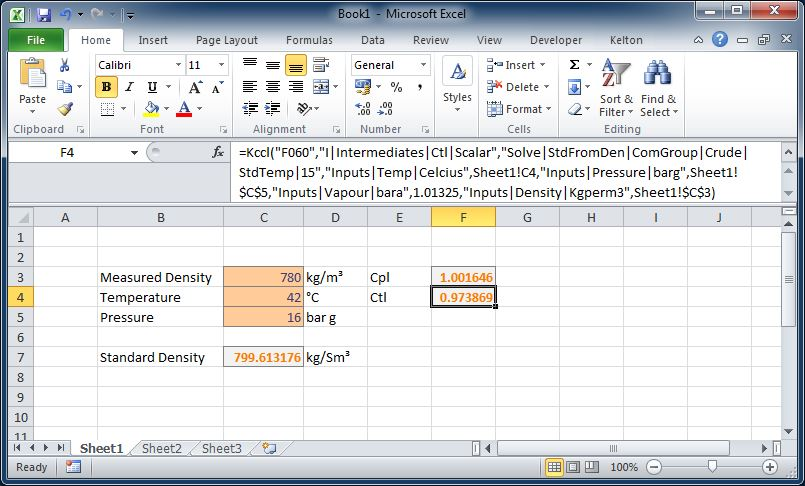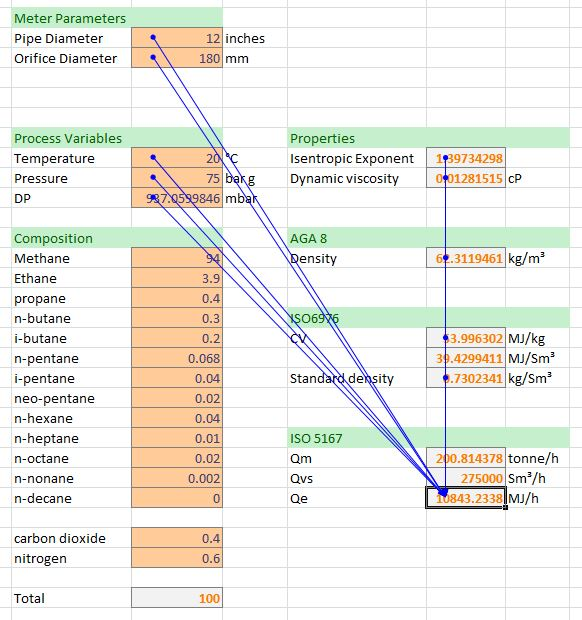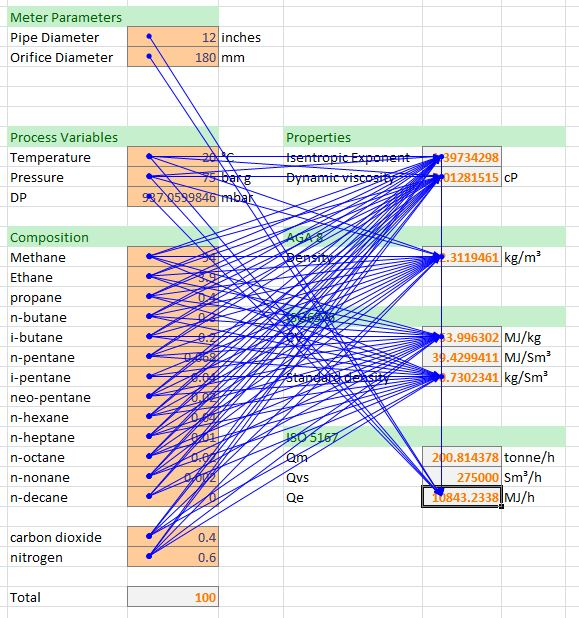# FloXL

FloXL makes FLOCALC calculations available as functions when using Microsoft Excel 2010. This feature is run from within Excel and there is no requirement to run FLOCALC to use FloXL.

In this example we would like to add a function to calculate standard density from the measured density, pressure and temperature using the 2004 API density referral calculation.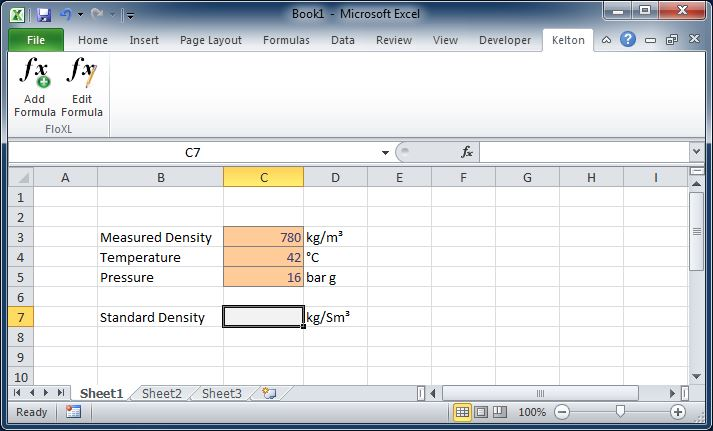To Add a FloXL function click on the Add Formula button on the Kelton ribbon; this will open a window which will let you select the appropriate calculation. As with FLOCALC the calculations are grouped for easy selection.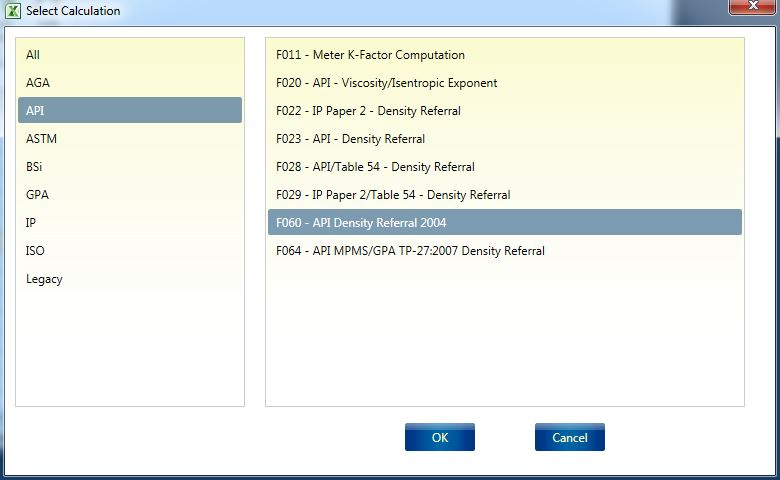## Configure Calculation

The FloXL calculation will open in a window similar to that when using a FLOCALC calculation.

### Options

Calculation options are set under the options tab; in this case we would like to calculate the standard density of crude oil using a standard temperature of 15 °C.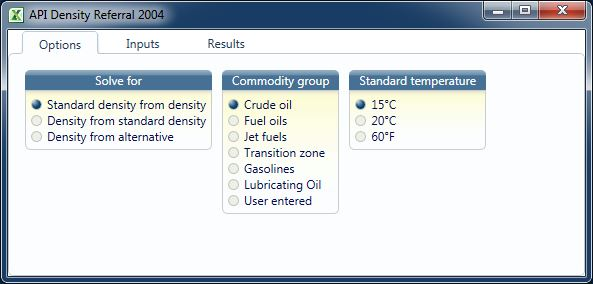### Inputs

When setting up the inputs the units must match those used in the spreadsheet otherwise the calculation will not return the correct result.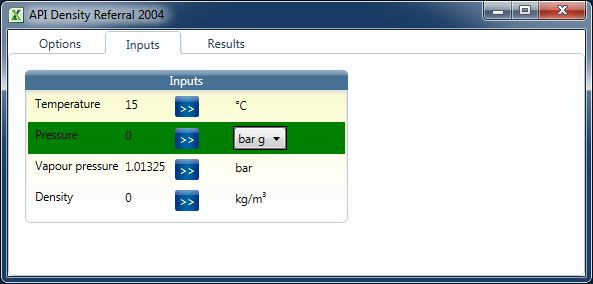There are a number of ways in which the input can be referenced to a cell in Excel.

Click and Select

To use this method, first click on the cell within Excel you would like to link and then click the button next to the parameter where you would like to use the value. This method works best when you are manipulate the windows on your screen to display the Excel workbook and the FloXL calculation window side by side.   Alternatively you can switch between windows by clicking on the Excel button on the taskbar at the bottom of the screen.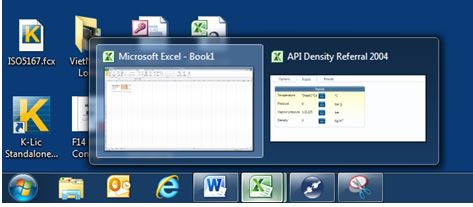Type the cell reference

The cell reference can be typed in place of the input value. Once the cell reference is entered it will be referenced to the Worksheet which is currently selected. As with any other Excel functions absolute and relative cell references can be used by preceding the Row numbers and Column letters with a \$.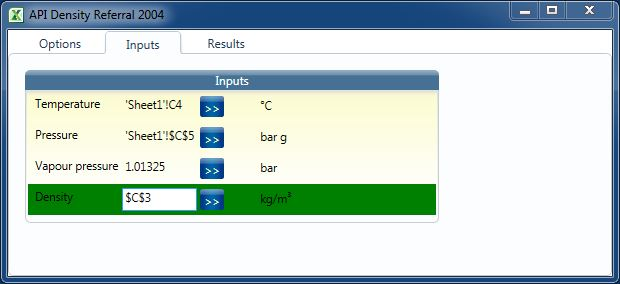Named cells

If you are familiar with the use of named cells within Excel this method works well with FloXL – Simply type the name of the cell in place of the cell reference.

### Results

Intermediates and outputs all appear in the results tab. The method of linking results to Excel is the same as when setting up inputs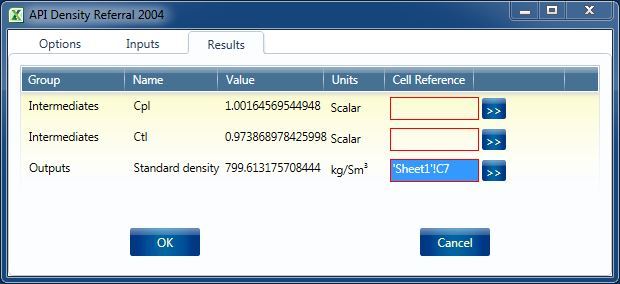## The Excel Function

Once you have configured the FloXL Function and clicked OK the Excel function will be entered in the cell which is referenced in the Results tab.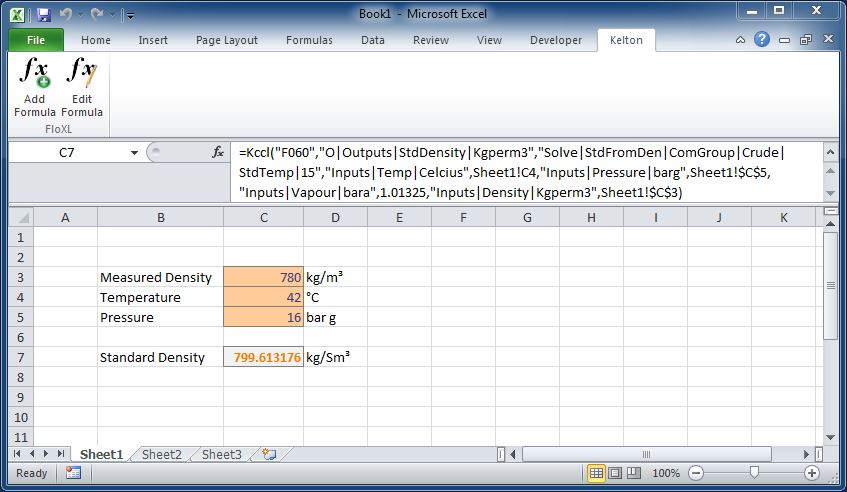To edit the function, click the edit formula button on the Kelton ribbon as FloXL functions use too many arguments to use the fx button in the formula bar. Functions will behave in the same way as a standard Excel function and can be copied, moved, dragged and dropped. This example has been shows the density which would result from changing the temperature of the oil: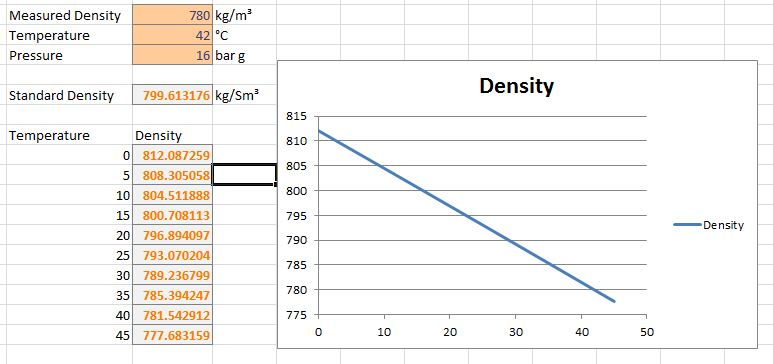## Multiple Outputs

A FloXL calculation may calculate a number of different parameters. In the example where we calculated standard density from measured density we also had the option of calculating Cpl and Ctl. Selecting multiple results will result in multiple Excel functions being created.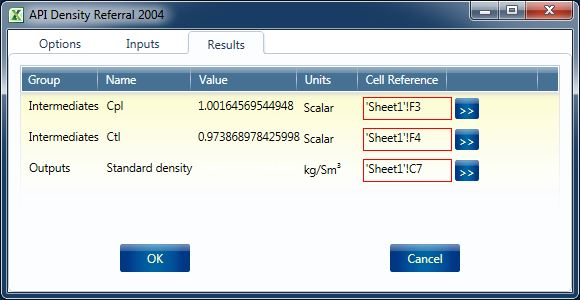It should be noted that the functions created are independent of each other and once created editing one function will not change the configuration of any function created at the same time.